# Choose a Colormap for your Plot#

This is an example of what colormaps are available in Py-ART, and how to add them to your own plots.

```print(__doc__)

# Author: Max Grover (mgrover@anl.gov)

import matplotlib.pyplot as plt
import numpy as np

import pyart
from pyart.testing import get_test_data
```

Plot the available colormaps

Let’s see which colormaps are available directly from Py-ART! We use a helper function from matplotlib to plot this.

```# Setup some helper functions and ranges to visualize our colormaps, from matplotlib

# Create figure and adjust figure height to number of colormaps
nrows = len(cmap_list)
figh = 0.35 + 0.15 + (nrows + (nrows - 1) * 0.1) * 0.22
fig, axs = plt.subplots(nrows=nrows, figsize=(6.4, figh))
fig.subplots_adjust(top=1 - 0.35 / figh, bottom=0.15 / figh, left=0.4, right=0.99)

axs.set_title(cmap_category + " Colormaps", fontsize=14)

for ax, cmap_name in zip(axs, cmap_list):
ax.text(
-0.01,
0.5,
f"pyart_{cmap_name}",
va="center",
ha="right",
fontsize=10,
transform=ax.transAxes,
)

# Turn off *all* ticks & spines, not just the ones with colormaps.
for ax in axs:
ax.set_axis_off()
```

Colorblind Friendly Colormaps

We recommend starting with these colorblind friendly colormaps. These colormaps are the most inclusive, and should be used where possible.

```plot_color_gradients(
"Colorblind Friendly",
["LangRainbow12", "HomeyerRainbow", "balance", "ChaseSpectral", "SpectralExtended"],
)
```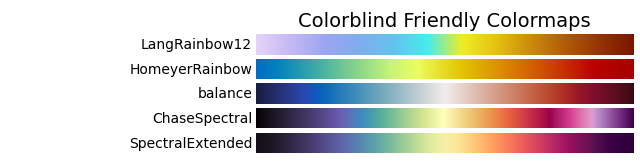Perceptually Uniform Colormaps

More generally, perceptually uniform colormaps are colormaps where the lightness value increases monotonically through the colormaps.

```plot_color_gradients(
"Sequential",
[
"Bu10",
"Bu7",
"Gray5",
"Gray9",
],
)
```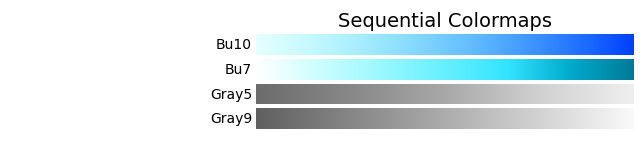Diverging Colormaps

Diverging colormaps are helpful when showing positive and negative values. This is when the 0 value is meaningful (ex. velocity)

```plot_color_gradients(
"Diverging",
[
"BlueBrown11",
"BrBu10",
"BrBu12",
"BuDOr12",
"BuDOr18",
"BuDRd12",
"BuDRd18",
"BuGr14",
"BuGy8",
"BuOr10",
"BuOr12",
"BuOr8",
"BuOrR14",
"GrMg16",
"RdYlBu11b",
],
)
```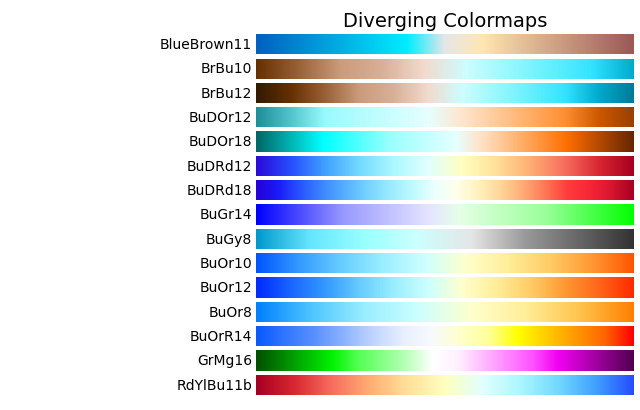Field-Specific Colormaps

There are some colormaps that useful for specific fields, such as “BlueBrown10” for terrain, or NWSRef for the National Weather Service reflectivity field

```plot_color_gradients(
"Field-specific ",
[
"BlueBrown10",
"Carbone11",
"Carbone17",
"Carbone42",
"Cat12",
"EWilson17",
"NWSRef",
"NWSVel",
"NWS_SPW",
"PD17",
"RRate11",
"RefDiff",
"SCook18",
"StepSeq25",
"SymGray12",
"Theodore16",
"Wild25",
],
)
```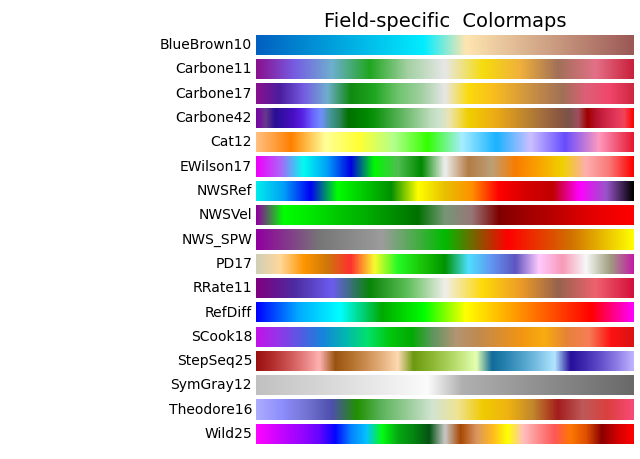Plot Using a Colormap from Matplotlib

Now, we can apply one of these colorbars to plot and compare to a colormap from matplotlib, starting with the matplotlib example.

```# Read in a sample cfradial file

# Setup a display to plot the data

# Start by plotting a regular matplotlib colormap (Spectral_r)
display.plot("reflectivity_horizontal", vmin=-32, vmax=64.0, cmap="Spectral_r")
```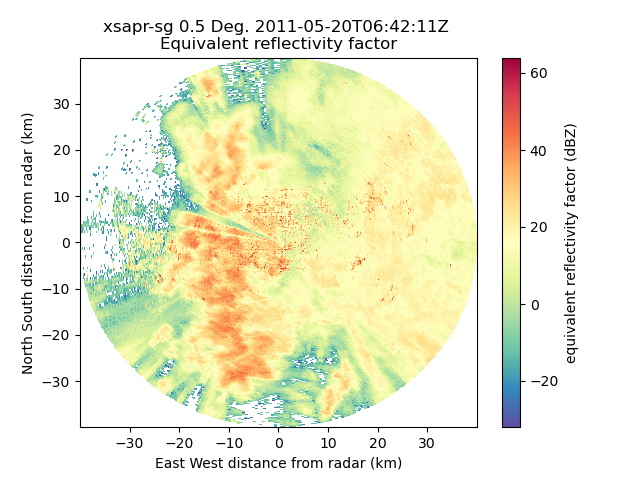Plot Using a Colormap from Py-ART

Let’s use one of our Py-ART’s colorbars now! We need to remember to add the pyart_ string in front of the colormap, as shown below. Setup a display to plot the data

```display = pyart.graph.RadarDisplay(radar)

# Start by plotting a regular matplotlib colormap (Spectral_r)
display.plot(
"reflectivity_horizontal", vmin=-32, vmax=64.0, cmap="pyart_HomeyerRainbow"
)
```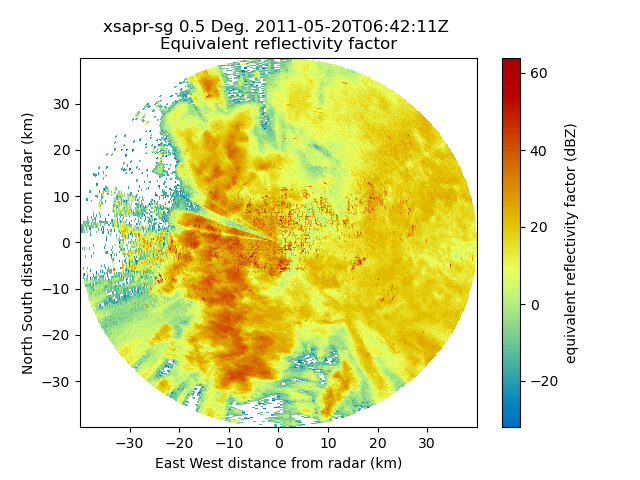Total running time of the script: ( 0 minutes 2.967 seconds)

Gallery generated by Sphinx-Gallery